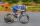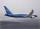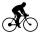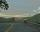# Velocity + multiplication - math problems

#### Number of problems found: 11

• CyclistA cyclist passes 88 km in 4 hours. How many kilometers did he pass in 8 hours?
• AircraftIf an airplane flies 846 km/h for 8 h, how far does it travel?
• Gear wheelsTwo gear wheels, which fit together, have the number of teeth z1=58 and z2=149. Calculate the speed of the first wheel, if the second wheel rotates 1232 revolutions per minute.
• HandsThe clock shows 12 hours. After how many minutes will angle between the hour and minute hand 90°? Consider the continuous movement of both hands hours.
• CyclingCyclist goes on a trip. First, drove 4 hours average speed 44 kilometers per hour, then slowed down and another 4 hours driving a speed 36 kilometers per hour. How many total kilometers cycling?
• Hiking tripRosie went on a hiking trip. The first day she walked 18kilometers. Each day since she walked 90 percent of what she walked the day before. What is the total distance Rosie has traveled by the end of the 10th day? Round your final answer to the nearest ki
• The cyclistThe cyclist went from village to town. The first half of the journey went at 20 km/h. The second half of the journey, which mostly fell, went at 39 km/h. All journey took 88 minutes. Calculate the distance from the village to the town.
• MovementFrom the crossing of two perpendicular roads started two cyclists (each at the different road). One runs at average speed 28 km/h, the second at average speed 24 km/h. Determine the distance between them after 45 minutes cycling.
• Motion problemFrom Levíc to Košíc go car at speed 81 km/h. From Košíc to Levíc go another car at speed 69 km/h. How many minutes before the meeting will be cars 27 km away?
• Bus vs. trainThe bus started from point A 10 minutes before the train started from the same place. The bus went an average speed of 49 km/h, train 77 km/h. To point B train and bus arrived simultaneously. Calculate the time of the train journey, if train and bus trave
• MotionIf you go at speed 3.7 km/h, you come to the station 42 minutes after leaving the train. If you go by bike to the station at speed 27 km/h, you come to the station 56 minutes before its departure. How far is the train station?

We apologize, but in this category are not a lot of examples.
Do you have an interesting mathematical word problem that you can't solve it? Submit a math problem, and we can try to solve it.

We will send a solution to your e-mail address. Solved examples are also published here. Please enter the e-mail correctly and check whether you don't have a full mailbox.

Please do not submit problems from current active competitions such as Mathematical Olympiad, correspondence seminars etc...
Do you want to convert velocity (speed) units? Velocity - math problems. Multiplication Problems.